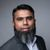Announcements
Applications are open for the 2024 Qlik Luminary Program. Apply by December 15 here.
cancel
Showing results for
Did you mean:Specialist III

## Sum of quantity where cdate >= d7

Dear All,

I would like to calculate sum of quantity where cdate >= d7.

I'm going to achieve my required result through following script and expression in Qlik Sense, but given below expression is returning Zero "0" value.

Please make correction, and share me expression.

My script:

temp:

date(Max([date/time])-6) as d7

Resident sales2015 Group By [date/time];

My expression:

sum({<cdate = {'>=[d7]'}>} quantity)

Kind regards,

Ishfaque Ahmed

1 Solution

Accepted SolutionsSpecialist III
Author

Dear All,

Problem has been resolved, here are the expressions.

1D = Sum({\$<[cdate] = {">=\$(=Date(Max([date/time]),'DD-MM-YY'))"}>} [quantity])

7D = Sum({\$<[cdate] = {">=\$(=Date(Max([date/time])-6,'DD-MM-YY'))"}>} [quantity])

15D = Sum({\$<[cdate] = {">=\$(=Date(Max([date/time])-15,'DD-MM-YY'))"}>} [quantity])

30D = Sum({\$<[cdate] = {">=\$(=Date(Max([date/time])-30,'DD-MM-YY'))"}>} [quantity])

Sum of Quantitiy - Expression

Kind regards,

Ishfaque Ahmed

10 RepliesMaster II

Hi,

Try to put this expression in a text box and see if  you are getting an output for this:

date(Max([date/time])-6)

Regards

KC

Best Regards,
KCNot applicable

Expand it with \$

like

sum({<cdate = {'>=\$(=[d7])'}>} quantity)

or even better

sum({<cdate = {'>=\$(=MAX([d7]))'}>} quantity)

so it works even  if d7 somehow  gets multiple values.Specialist III
Author

Dear Jyothish,

Max values is coming but not working in Qlik Sense Expression.

Kind regards,

Ishfaque AhmedSpecialist III
Author

Dear Edral,

Your both expressions are not working.

Kind regards,

Ishfaque AhmedAnonymous
Not applicable

You will need to use double quotes to induce the search functionality.

sum({<cdate = {">=\$(=[d7])"}>} quantity)Not applicable

I am sure syntax is correct even more correct with double quotes as Chris suggested.

here is a file with the syntax,

MaxDate.qvf

The problem is related with date format and specifically the minus operation.

enclose minus operation within Parentheses so that Date format applies correctly.Specialist III
Author

Dear All,

Kindly find attached my Qlik Sense App. Please make correction.

Kind regards,

Ishfaque AhmedPartner - Champion III

Hi

I think the problem comes down to the Group By in the script in your original post.The group by means that there are multiple values for d7 which is why your code does not work.Your script should read:

temp:

Max([date/time]) - 6 as d7

Resident sales2015;

I usually then put the field into a variable in the script:

Let vd7= Peek('d7');

sum({<cdate = {">=\$(=Date(vd7))"}>} quantity)

(I am assuming that you need dates greater than Max([Date/time])

HTH

Jonathan

Logic will get you from a to b. Imagination will take you everywhere. - A EinsteinSpecialist III
Author

Dear Jonathan,

Your given solution is not working even I applied in another app.

My app is attached, please check.

In this time, I made expression for field "1D". Value should be 1 for 1D but It's coming 0.

Kind regards,

Ishfaque AhmedTags
Community Browser